# Virtual COMSATS Inferential Statistics Lecture10 Ossam Chohan Assistant

• Slides: 71Virtual COMSATS Inferential Statistics Lecture-10 Ossam Chohan Assistant Professor CIIT Abbottabad 1Recap of previous lecture • We discussed confidence intervals • How to calculate critical value using z table • How to understand problem with respect to the cases. 2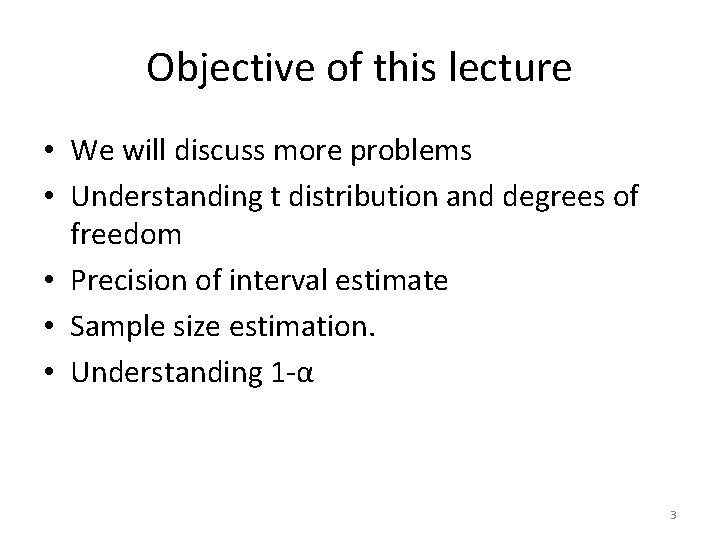Objective of this lecture • We will discuss more problems • Understanding t distribution and degrees of freedom • Precision of interval estimate • Sample size estimation. • Understanding 1 -α 3Problem-8 • A sample poll of 100 voters chosen at random from all voters in a given district indicated that 55% of them were in favor of a particular candidate. Find the – 95% CI for the proportion of all the voters in favor of this candidate. – 99. 73% confidence limits for the proportion of all the voters in favor of this candidate. 4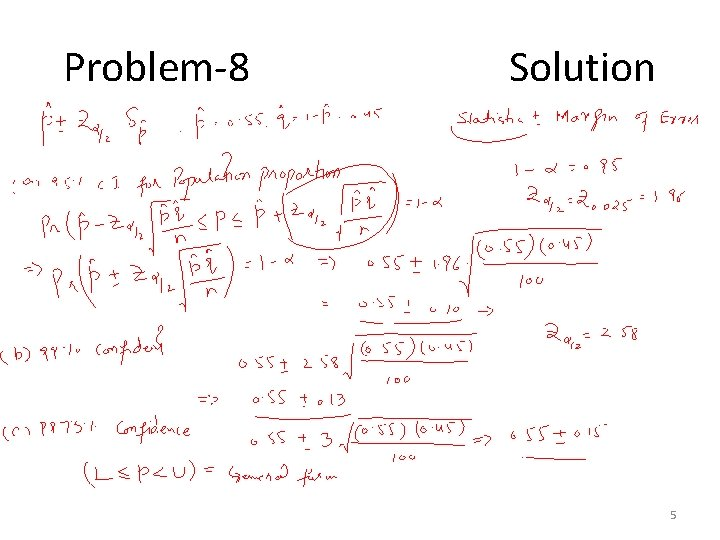Problem-8 Solution 5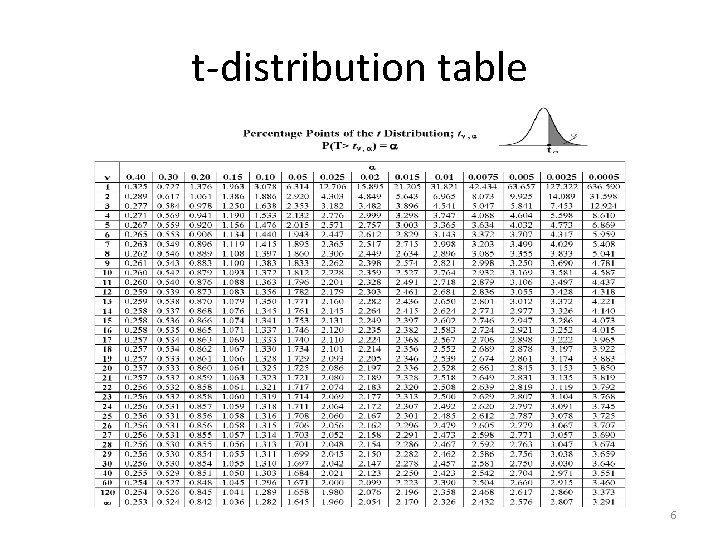t-distribution table 6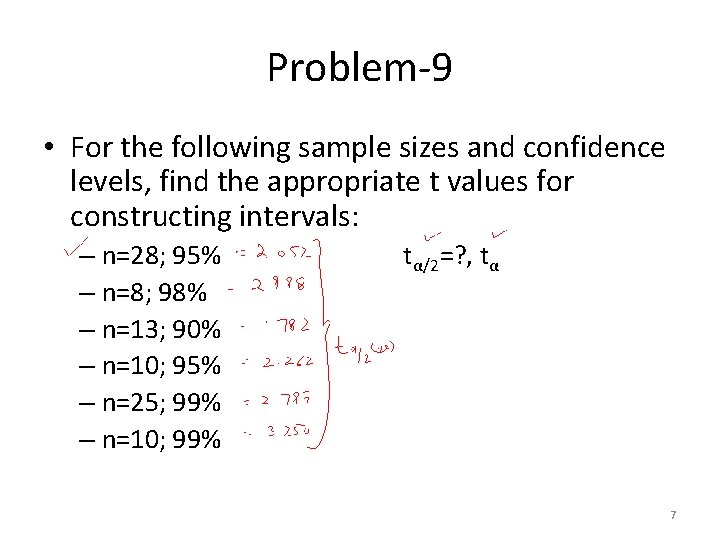Problem-9 • For the following sample sizes and confidence levels, find the appropriate t values for constructing intervals: – n=28; 95% – n=8; 98% – n=13; 90% – n=10; 95% – n=25; 99% – n=10; 99% tα/2=? , tα 7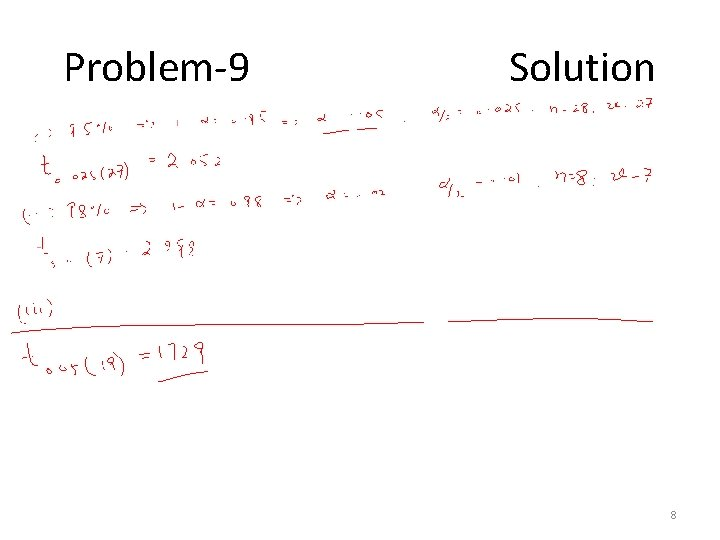Problem-9 Solution 8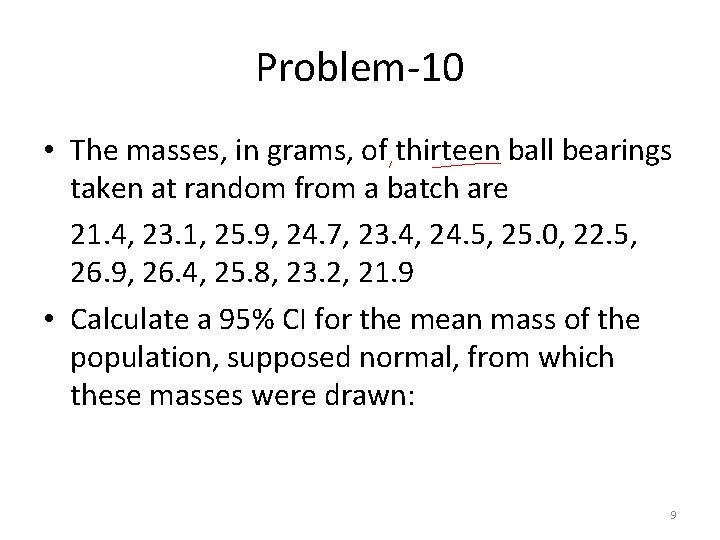Problem-10 • The masses, in grams, of thirteen ball bearings taken at random from a batch are 21. 4, 23. 1, 25. 9, 24. 7, 23. 4, 24. 5, 25. 0, 22. 5, 26. 9, 26. 4, 25. 8, 23. 2, 21. 9 • Calculate a 95% CI for the mean mass of the population, supposed normal, from which these masses were drawn: 9Problem-10 Solution • • • Sample mean= 314. 7/13= 24. 21 s= √[Σ(xi- )2]/n-1 s= √ 3. 12= 1. 77 v=n-1, degree of freedom 1 -α=0. 95, α=0. 05 tα/2(v)= t 0. 025(12)=2. 179 10Problem-10 Solution 11Problem-11 • The following sample of eight observations is from an infinite population with normal distribution: 75. 3, 76. 4, 83. 2, 91. 0, 80. 1, 77. 5, 84. 8, 81 • Find the sample mean. • Estimate the population standard deviation and standard error. • Construct a 98% CI for the population mean. 12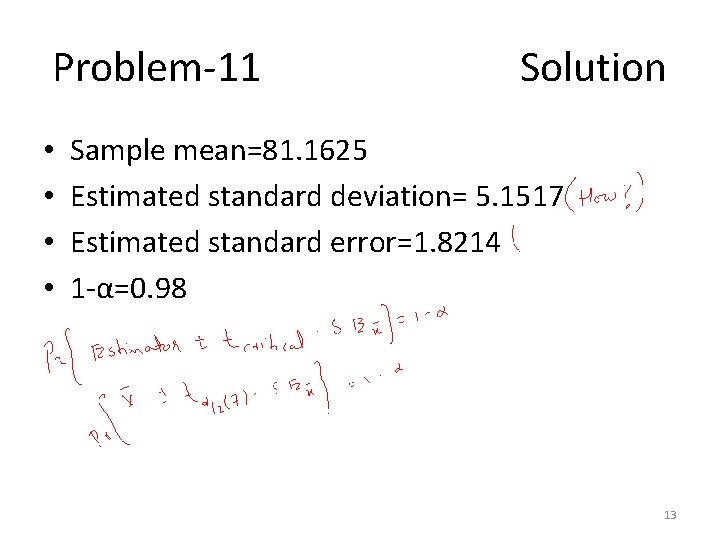Problem-11 Solution • • Sample mean=81. 1625 Estimated standard deviation= 5. 1517 Estimated standard error=1. 8214 1 -α=0. 98 13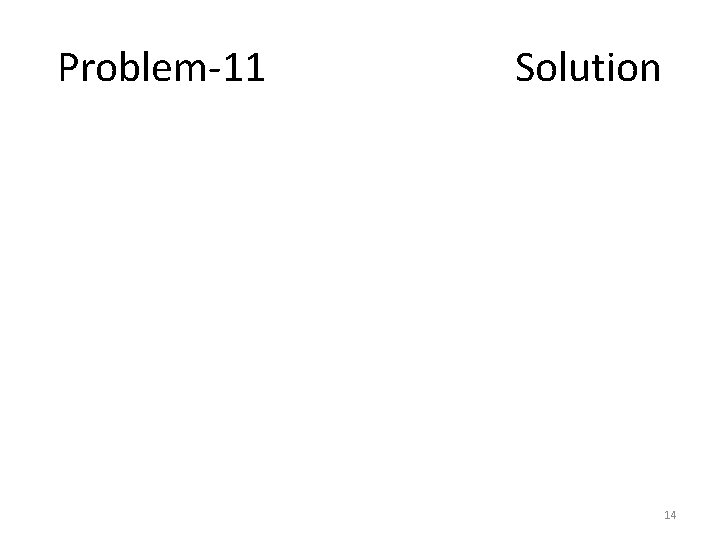Problem-11 Solution 14Problem-12 • Twelve bank tellers were randomly sampled and it was determined they made an average of 3. 6 errors per day with a sample of standard deviation of 0. 42 error. Construct a 90% CI for population mean of errors per day. 15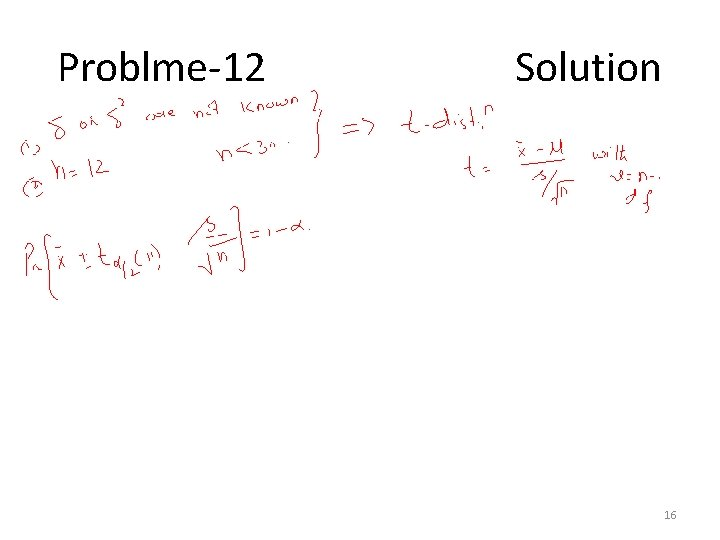Problme-12 Solution 16Problem-13 • In a certain large city, a random sample of 400 families contacted by a local TV station showed that 275 owned color TV sets. Find an approximate 90% confidence interval on that true proportion of all families living in the city who – Own color TV sets – Don’t have color TV sets 17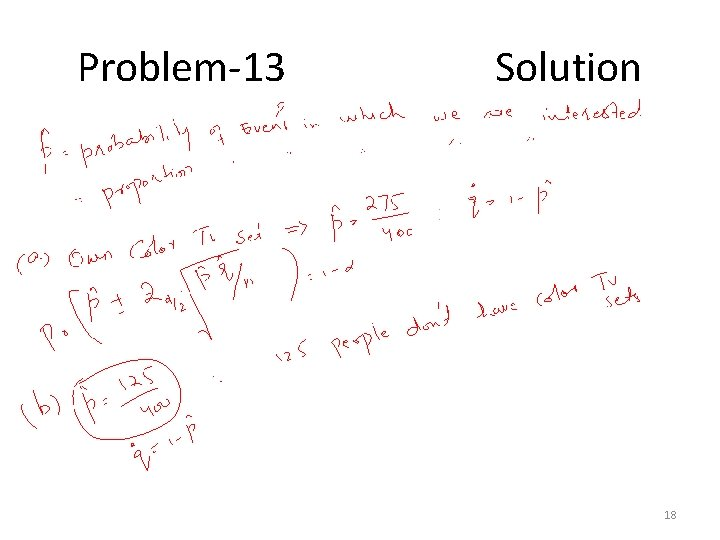Problem-13 Solution 18Problem-14 • The average monthly electricity consumption for a sample of 100 families is 1250 units. Assuming the standard deviation of electric consumption of all families is 150 units, construct a 95% Confidence Interval estimate of the actual mean electric consumption. 19Problem-14 Solution 20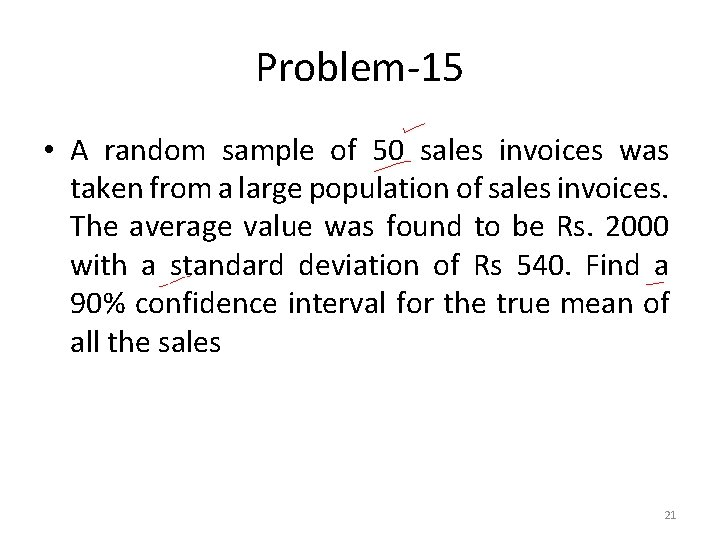Problem-15 • A random sample of 50 sales invoices was taken from a large population of sales invoices. The average value was found to be Rs. 2000 with a standard deviation of Rs 540. Find a 90% confidence interval for the true mean of all the sales 21Problem-15 Solution 22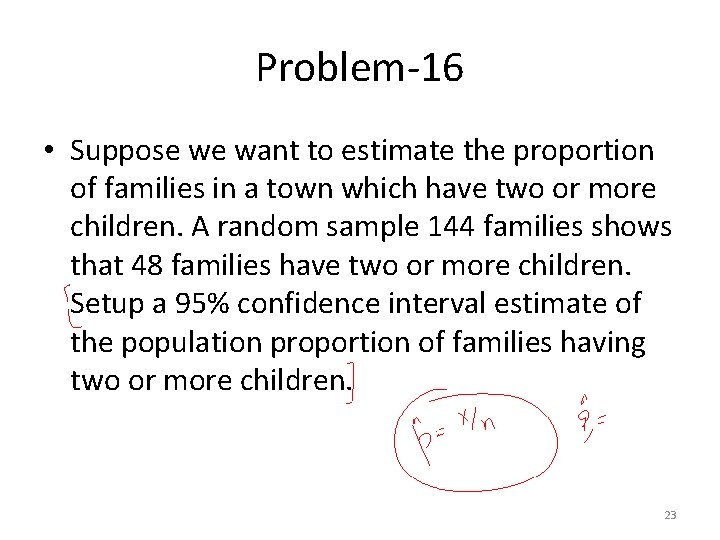Problem-16 • Suppose we want to estimate the proportion of families in a town which have two or more children. A random sample 144 families shows that 48 families have two or more children. Setup a 95% confidence interval estimate of the population proportion of families having two or more children. 23Problem-16 Solution 24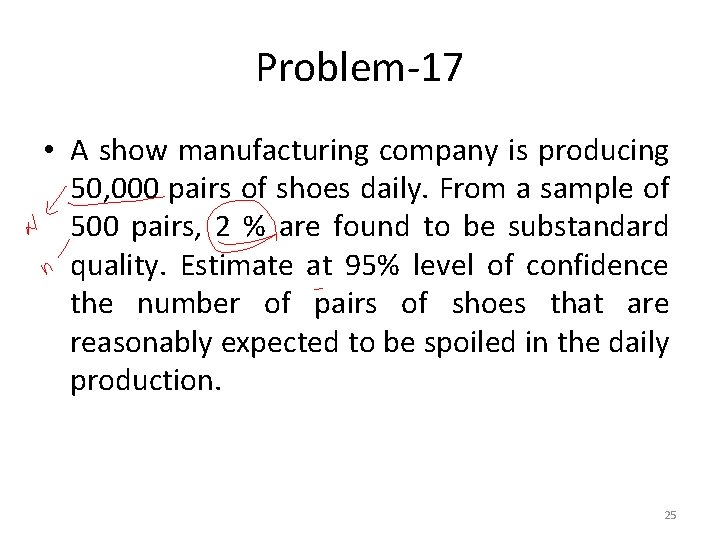Problem-17 • A show manufacturing company is producing 50, 000 pairs of shoes daily. From a sample of 500 pairs, 2 % are found to be substandard quality. Estimate at 95% level of confidence the number of pairs of shoes that are reasonably expected to be spoiled in the daily production. 25Problem-17 Solution 26Estimating Sample Size • Estimation of sample size is important to infer about population parameter. • Standard errors are generally inversely proportional to sample size. • It means that n is also related to width of confidence interval. 27Precision of confidence interval • The precision with which a confidence interval estimates the true population parameters is determined by the width of the confidence interval. • Narrow the CI, more precise the estimate and vice versa. • Width of CI depends upon – Specified level of confidence – Sample size – Population standard deviation 28• More precision can be achieved by increasing sample size. • But cost of increasing sample size, sometimes not possible. • Therefore to achieve desired precision, lower the confidence. • Lets have a look on some problems. 29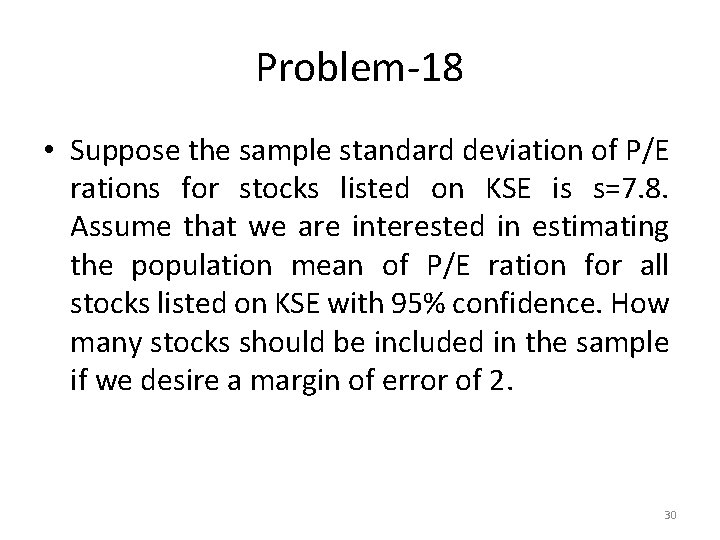Problem-18 • Suppose the sample standard deviation of P/E rations for stocks listed on KSE is s=7. 8. Assume that we are interested in estimating the population mean of P/E ration for all stocks listed on KSE with 95% confidence. How many stocks should be included in the sample if we desire a margin of error of 2. 30Problem-18 Solution 31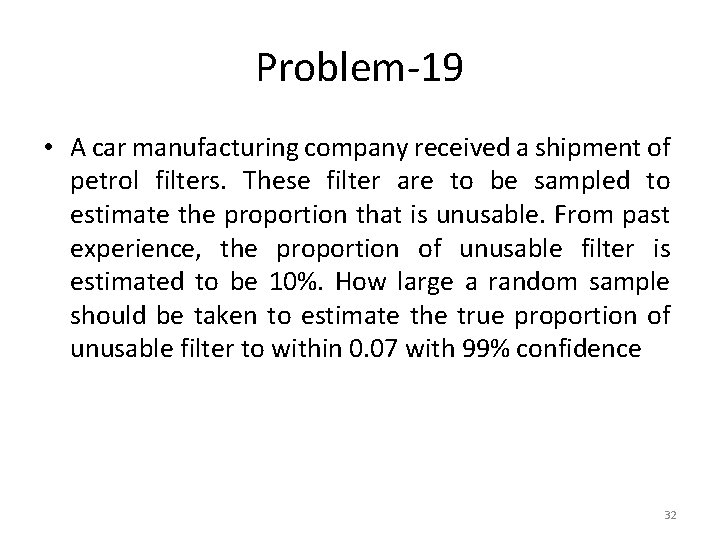Problem-19 • A car manufacturing company received a shipment of petrol filters. These filter are to be sampled to estimate the proportion that is unusable. From past experience, the proportion of unusable filter is estimated to be 10%. How large a random sample should be taken to estimate the true proportion of unusable filter to within 0. 07 with 99% confidence 32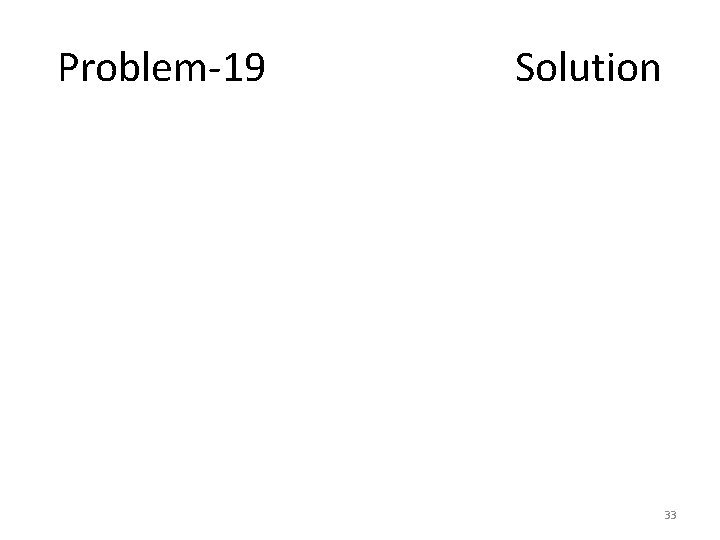Problem-19 Solution 33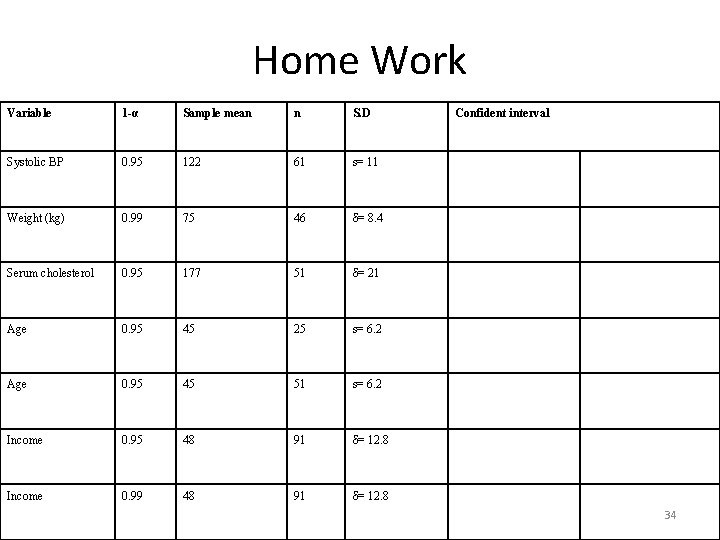Home Work Variable 1 -α Sample mean n S. D Systolic BP 0. 95 122 61 s= 11 Weight (kg) 0. 99 75 46 δ= 8. 4 Serum cholesterol 0. 95 177 51 δ= 21 Age 0. 95 45 25 s= 6. 2 Age 0. 95 45 51 s= 6. 2 Income 0. 95 48 91 δ= 12. 8 Income 0. 99 48 91 δ= 12. 8 Confident interval 34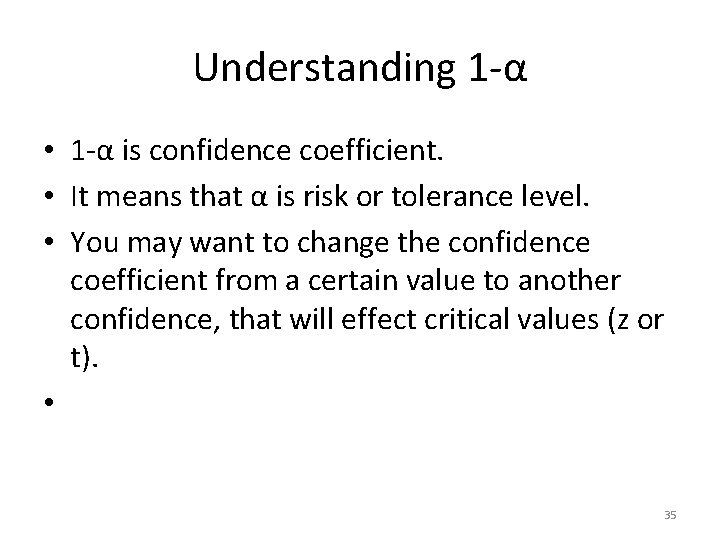Understanding 1 -α • 1 -α is confidence coefficient. • It means that α is risk or tolerance level. • You may want to change the confidence coefficient from a certain value to another confidence, that will effect critical values (z or t). • 35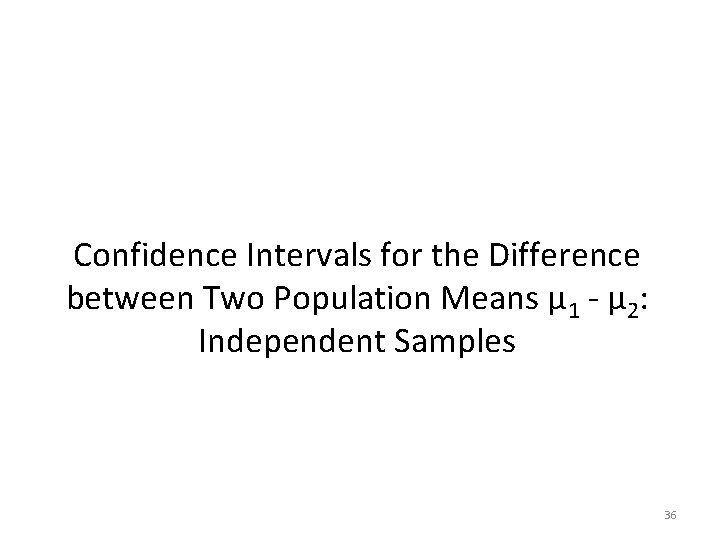Confidence Intervals for the Difference between Two Population Means µ 1 - µ 2: Independent Samples 36Confidence Intervals for the Difference between Two Population Means µ 1 - µ 2: Independent Samples • Two random samples are drawn from the two populations of interest. • Because we compare two population means, we use the statistic . 37Estimating the Difference Between Two Population Means The estimates of the population parameters are calculated from the sample data. Properties of the Sampling Distribution of , the Difference Between Two Sample Means: • When independent random sample of n 1 and n 2 have been selected from populations with means m 1 and m 2 and variances, respectively, the sampling distribution of the differences has the following properties: 1. The mean and the standard error of are and 382. If the sampled populations are normally distributed, then the sampling distribution of is exactly normally distributed, regardless of the sample size. 3. If the sampled populations are not normally distributed, then the sampling distribution of is approximately normally distributed when n 1 and n 2 are large, due to the CLT. • Since m 1 m 2 is the mean of the sampling distribution, is an unbiased estimator of (m 1 m 2 ) with an approximately normal distribution. • The statistic has an approximately standard normal z distribution. © 1998 Brooks/Cole Publishing/ITP 39Point Estimation of (m 1 m 2 ) : Point estimator: Margin of error: • If are unknown, but both n 1 and n 2 are 30 or more, you can use the sample variances to estimate A (1 a )100% Confidence Interval for (m 1 m 2 ) : © 1998 Brooks/Cole Publishing/ITP 40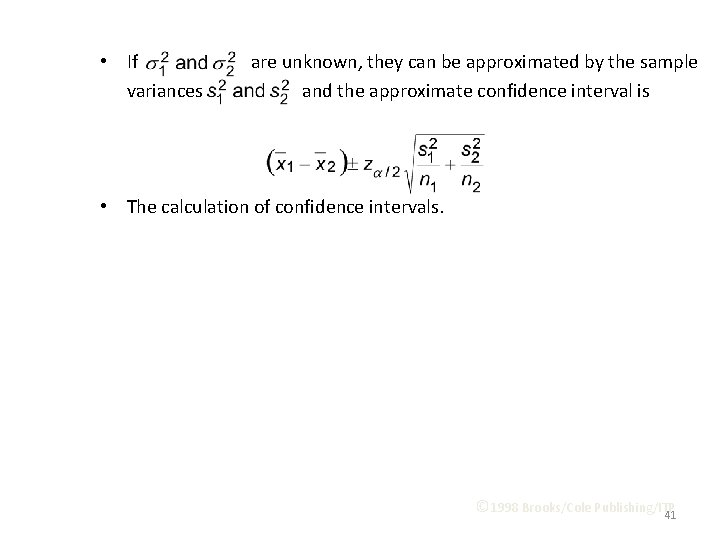• If are unknown, they can be approximated by the sample variances and the approximate confidence interval is • The calculation of confidence intervals. © 1998 Brooks/Cole Publishing/ITP 41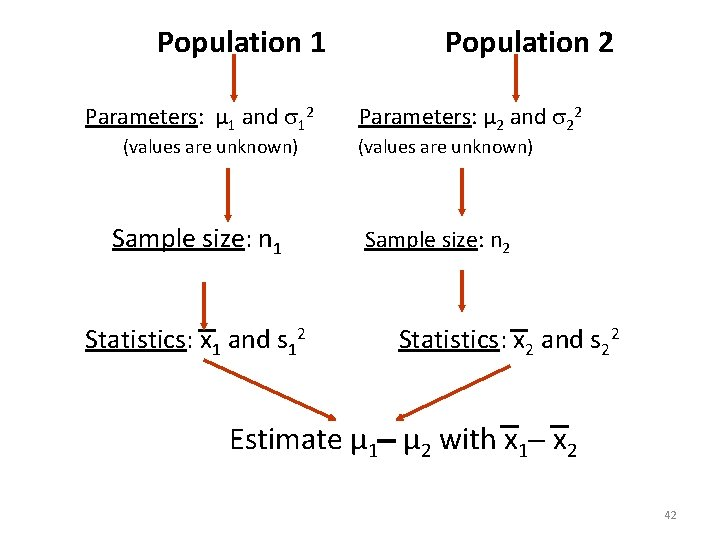Population 1 Population 2 Parameters: µ 1 and 12 Parameters: µ 2 and 22 (values are unknown) Sample size: n 1 Sample size: n 2 Statistics: x 1 and s 12 Statistics: x 2 and s 22 Estimate µ 1 µ 2 with x 1 x 2 4243Confidence Interval for m 1 – m 2 What about the conditions? What if unknown variances are equal? ? ? 44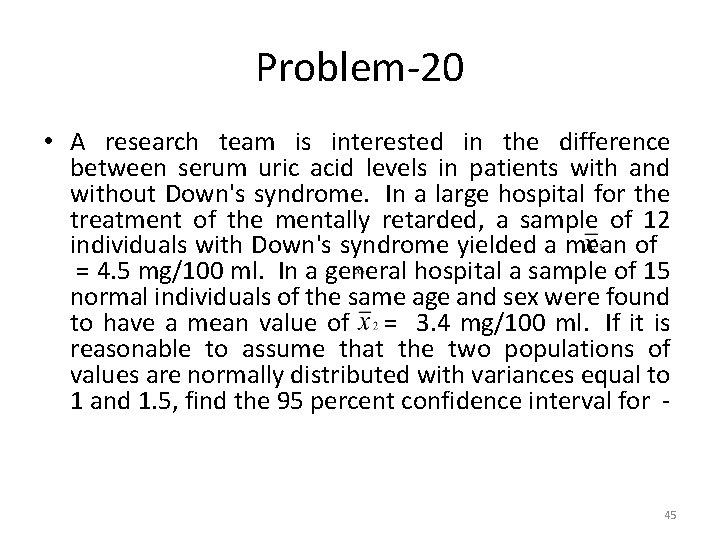Problem-20 • A research team is interested in the difference between serum uric acid levels in patients with and without Down's syndrome. In a large hospital for the treatment of the mentally retarded, a sample of 12 individuals with Down's syndrome yielded a mean of = 4. 5 mg/100 ml. In a general hospital a sample of 15 normal individuals of the same age and sex were found to have a mean value of = 3. 4 mg/100 ml. If it is reasonable to assume that the two populations of values are normally distributed with variances equal to 1 and 1. 5, find the 95 percent confidence interval for - 45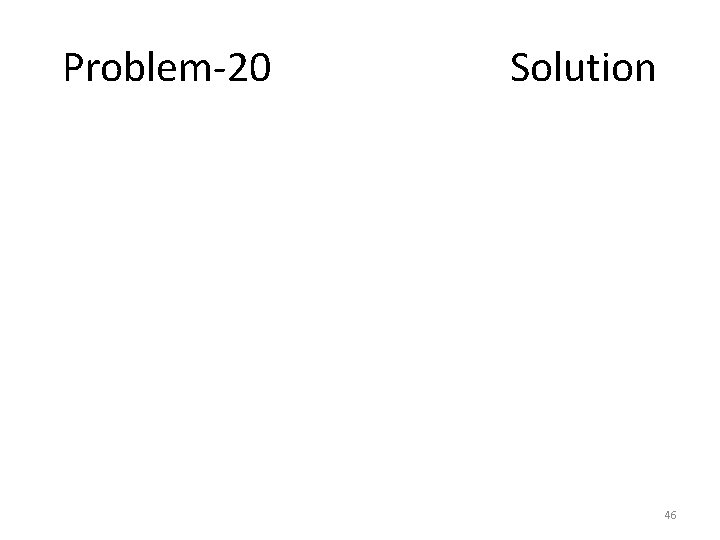Problem-20 Solution 46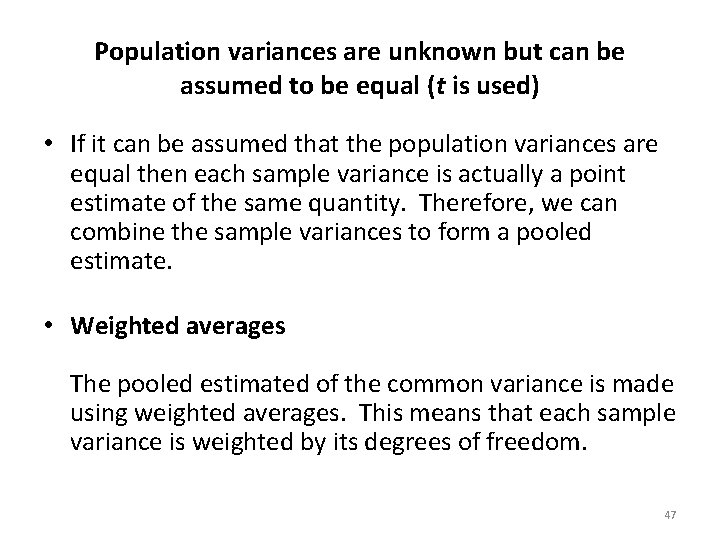Population variances are unknown but can be assumed to be equal (t is used) • If it can be assumed that the population variances are equal then each sample variance is actually a point estimate of the same quantity. Therefore, we can combine the sample variances to form a pooled estimate. • Weighted averages The pooled estimated of the common variance is made using weighted averages. This means that each sample variance is weighted by its degrees of freedom. 47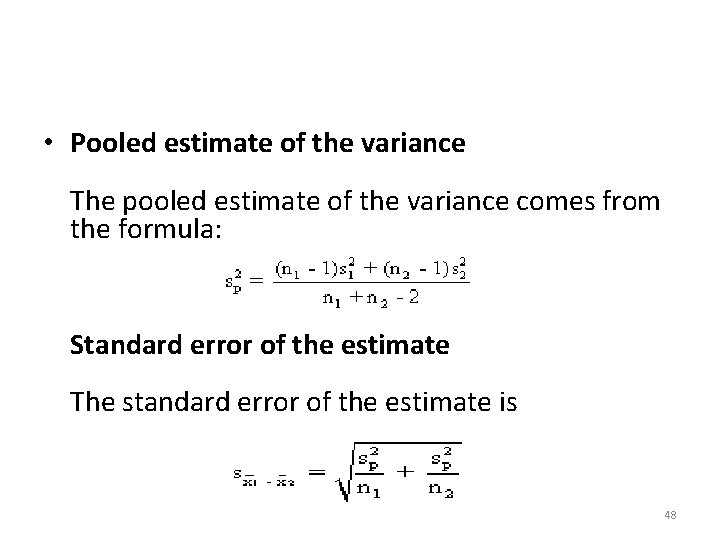• Pooled estimate of the variance The pooled estimate of the variance comes from the formula: Standard error of the estimate The standard error of the estimate is 48Confidence interval The 100(1 - ) confidence interval for µ 1 -µ 2 is 49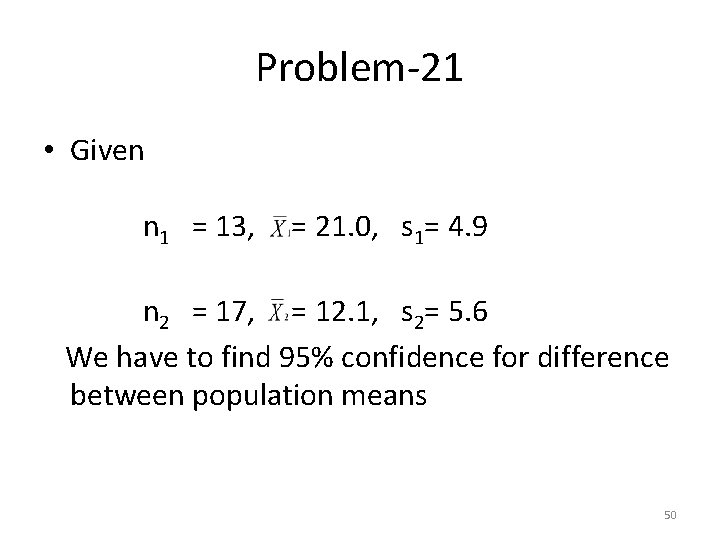Problem-21 • Given n 1 = 13, = 21. 0, s 1= 4. 9 n 2 = 17, = 12. 1, s 2= 5. 6 We have to find 95% confidence for difference between population means 505152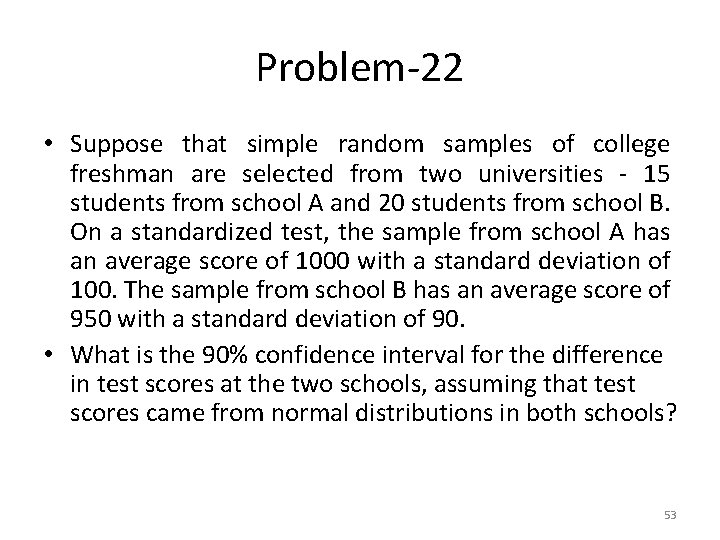Problem-22 • Suppose that simple random samples of college freshman are selected from two universities - 15 students from school A and 20 students from school B. On a standardized test, the sample from school A has an average score of 1000 with a standard deviation of 100. The sample from school B has an average score of 950 with a standard deviation of 90. • What is the 90% confidence interval for the difference in test scores at the two schools, assuming that test scores came from normal distributions in both schools? 53Problem-22 Solution 54Problem-23 • Suppose the Cartoon Network conducts a nation-wide survey to assess viewer attitudes toward Superman. Using a simple random sample, they select 400 boys and 300 girls to participate in the study. Forty percent of the boys say that Superman is their favorite character, compared to thirty percent of the girls. What is the 90% confidence interval for the true difference in attitudes toward Superman? 55Problem-23 Discussion • The sampling method must be simple random sampling. This condition is satisfied; the problem statement says that we used simple random sampling. • Both samples should be independent. This condition is satisfied since neither sample was affected by responses of the other sample. • The sampling distribution should be approximately normally distributed. Because each sample size is large, we know from the central limit theorem that the sampling distribution of the difference between sample proportions will be normal or nearly normal; so this condition is satisfied. 56Problem-23 Solution 57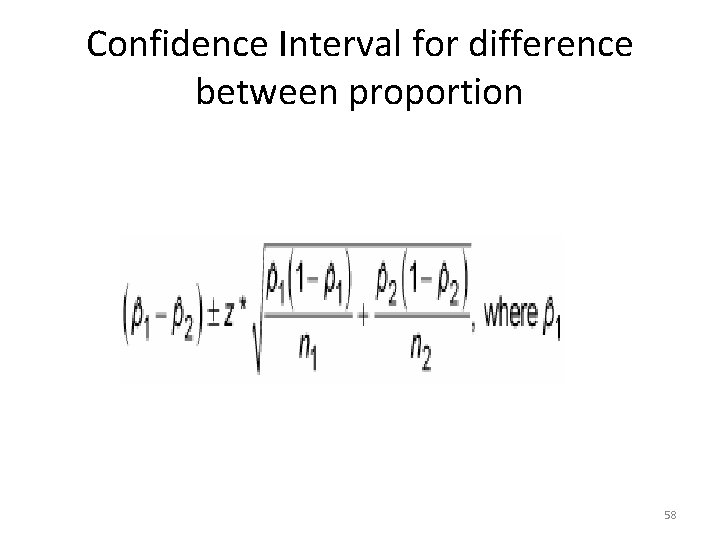Confidence Interval for difference between proportion 58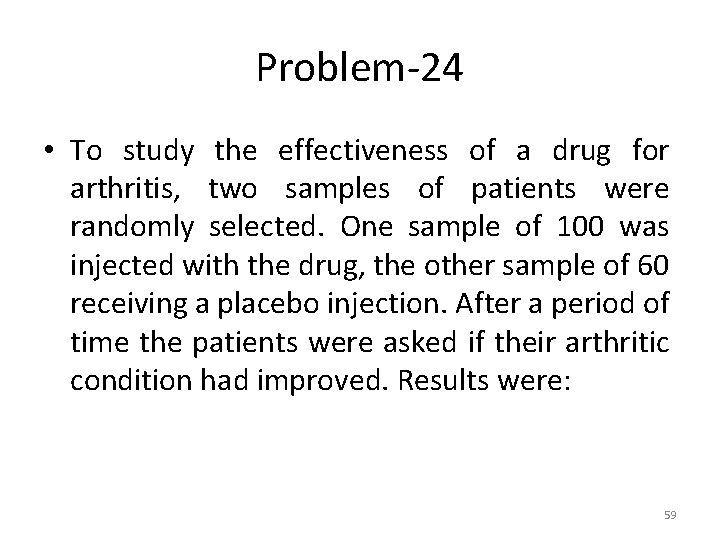Problem-24 • To study the effectiveness of a drug for arthritis, two samples of patients were randomly selected. One sample of 100 was injected with the drug, the other sample of 60 receiving a placebo injection. After a period of time the patients were asked if their arthritic condition had improved. Results were: 59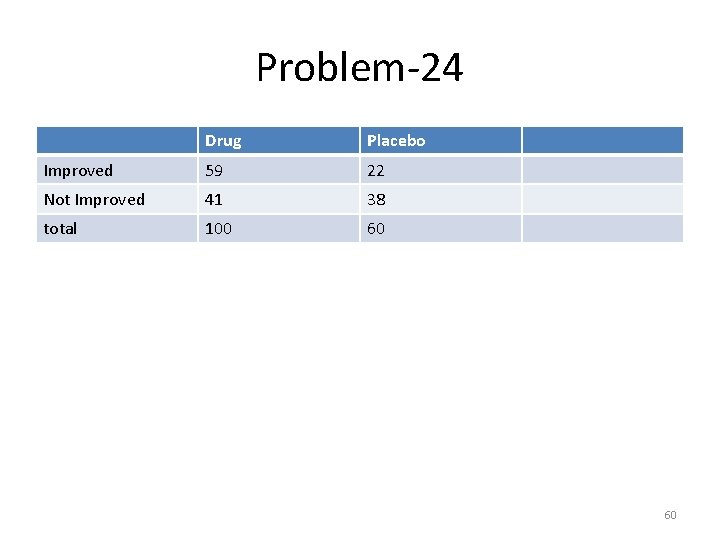Problem-24 Drug Placebo Improved 59 22 Not Improved 41 38 total 100 60 60Problem-24 Solution 61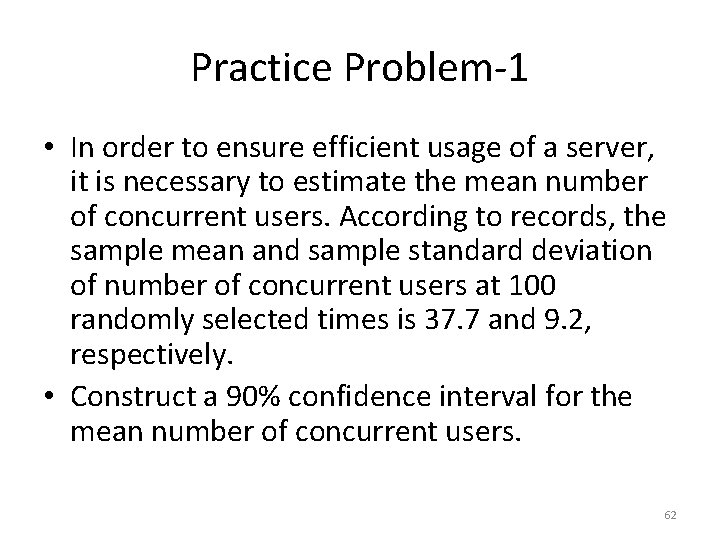Practice Problem-1 • In order to ensure efficient usage of a server, it is necessary to estimate the mean number of concurrent users. According to records, the sample mean and sample standard deviation of number of concurrent users at 100 randomly selected times is 37. 7 and 9. 2, respectively. • Construct a 90% confidence interval for the mean number of concurrent users. 62Practice Problem-2 • To assess the accuracy of a laboratory scale, a standard weight that is known to weigh 1 gram is repeatedly weighed 4 times. The resulting measurements (in grams) are: 0. 95, 1. 02, 1. 01, 0. 98. Assume that the weighing by the scale when the true weight is 1 gram are normally distributed with mean. • Use these data to compute a 95% confidence interval for µ. 63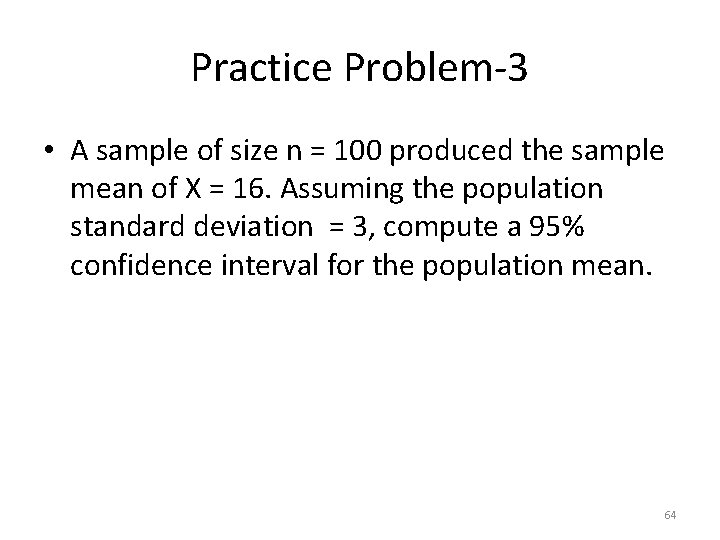Practice Problem-3 • A sample of size n = 100 produced the sample mean of X = 16. Assuming the population standard deviation = 3, compute a 95% confidence interval for the population mean. 64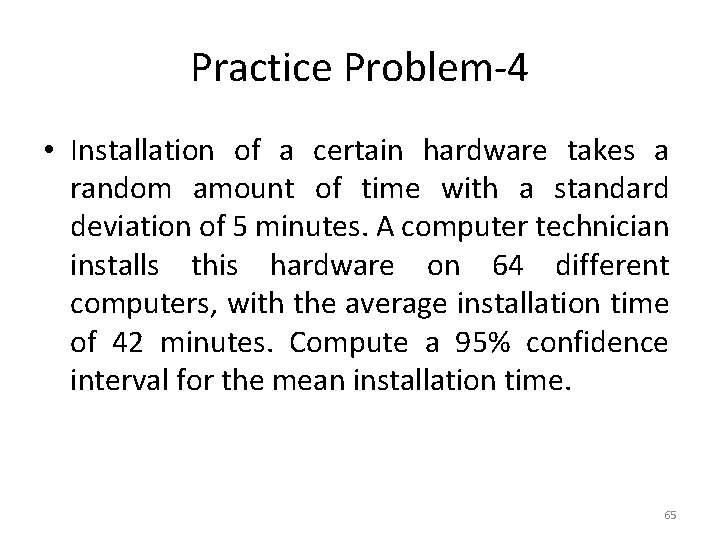Practice Problem-4 • Installation of a certain hardware takes a random amount of time with a standard deviation of 5 minutes. A computer technician installs this hardware on 64 different computers, with the average installation time of 42 minutes. Compute a 95% confidence interval for the mean installation time. 65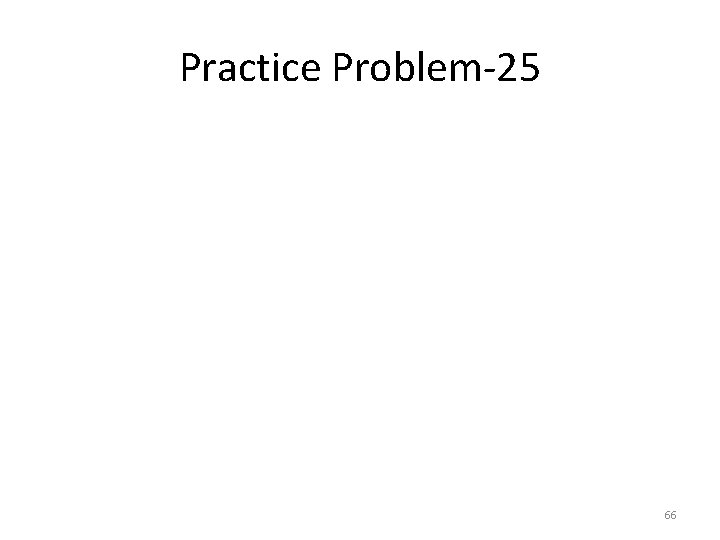Practice Problem-25 66Example: confidence interval for m 1 – m 2 • Example – Do people who eat high-fiber cereal for breakfast consume, on average, fewer calories for lunch than people who do not eat highfiber cereal for breakfast? – A sample of 150 people was randomly drawn. Each person was identified as a consumer or a non-consumer of high-fiber cereal. – For each person the number of calories consumed at lunch was recorded. 67Common Mistake !!! A common mistake is to calculate a one-sample confidence interval for m 1, a one-sample confidence interval for m 2, and to then conclude that m 1 and m 2 are equal if the confidence intervals overlap. This is WRONG because the variability in the sampling distribution for from two independent samples is more complex and must take into account variability coming from both samples. Hence the more complex formula for the standard error. 68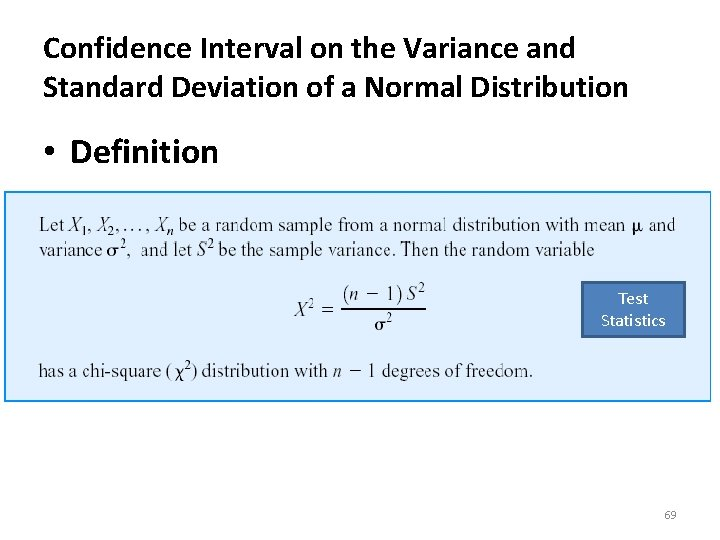Confidence Interval on the Variance and Standard Deviation of a Normal Distribution • Definition Test Statistics 69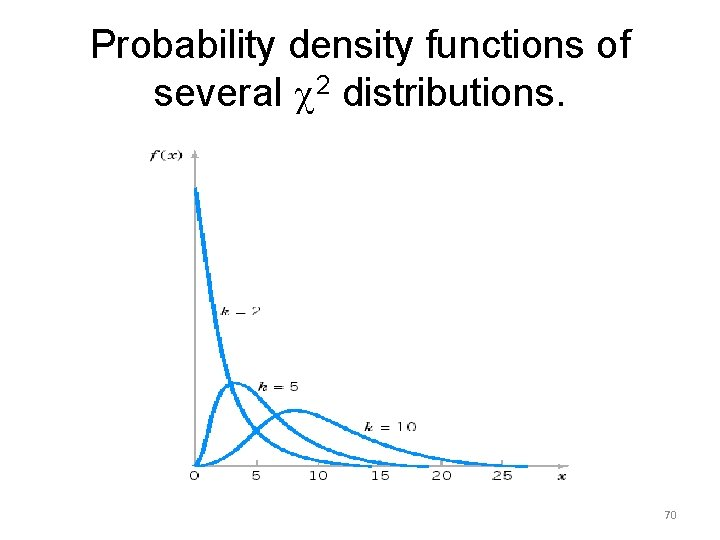Probability density functions of several 2 distributions. 7071